The default emphasis in the Fit Model launch window is based on the number of rows, n, the number of effects (k) entered in the Construct Model Effects list, and the attributes applied to effects.
 • If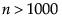, the Emphasis is set to Minimal Report.
 • Ifand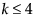, the Emphasis is set at Effect Leverage
 • If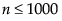and, the Emphasis is set at Effect Screening.
 • If any effect has a Random Effect attribute, the Emphasis is set to Minimal Report.
 • If none of these conditions hold, the Emphasis is set at Effect Screening.
In Example of a Custom Test, you are interested in testing three contrasts using the Cholesterol.jmp sample data table. Specifically, you want to compare:
 • the mean responses for treatments A and B,
 • the mean response for treatments A and B combined to the mean response for the control group,
 • the mean response for treatments A and B combined to the mean response for the combined control and placebo groups.
To derive the contrast coefficients that you enter into the Custom Test columns, do the following. Denote the theoretical effects for the four treatment groups as: αA, αB, αControl, and αPlacebo. These are the treatment effects, so they are constrained to sum to 0. Because the parameters associated with the indicator variables represent only the first three effects, you need to formulate your contrasts in terms of these first three effects. See Details of Custom Test Example and Interpretation of Parameters in Statistical Details for more information.
 • Compare treatment A to treatment B: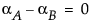• Compare treatments A and B to the control group: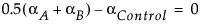• Compare treatments A and B to the control and placebo groups:
Consider a data set with n observations and p - 1 predictors. Define the matrix X to be the design matrix. That is, X is the n by p matrix whose first column consists of 1s and whose remaining p - 1 columns consist of the p - 1 predictor values. (Nominal columns are coded in terms of indicator predictors. Each of these is a column in the matrix X.)
where Y represents the vector of response values.
The correlation matrix for the estimates is obtained by dividing each entry in the covariance matrix by the product of the square roots of the diagonal entries. Define V to be the diagonal matrix whose entries are the square roots of the diagonal entries of the covariance matrix:
Effect leverage plots are also referred to as partial-regression residual leverage plots (Belsley, Kuh, and Welsch, 1980) or added variable plots (Cook and Weisberg, 1982). Sall (1990) generalized these plots to apply to any linear hypothesis.
 • Effect Leverage plots show observations relative to the hypothesis that the effect is not in the model, given that all other effects are in the model.
 • The Whole Model leverage plot, given in the Actual by Predicted Plot report, shows the observations relative to the hypothesis of no factor effects.
For each observation, consider the point with x-axis value vx and y-axis value vy where:
 • vx is the constrained residual minus the unconstrained residual, r0 - r, reflecting information left over once the constraint is applied
 • vy is the x-axis value plus the unconstrained residual
Construction of Leverage Plot
where x = [1 x] is the 2-vector of predictors.
 • Significant: If the slope parameter is significantly different from zero, the confidence curves cross the horizontal line at the response mean.
 • Borderline: If the t test for the slope parameter is sitting right on the margin of significance, the confidence curve is asymptotic to the horizontal line at the response mean.
 • Not Significant: If the slope parameter is not significantly different from zero, the confidence curve does not cross the horizontal line at the response mean.
Upper(z) =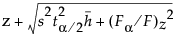Lower(z) =where F is the F statistic for the hypothesis and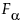is the reference value for significance α. And, where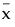is a row vector consisting of suitable middle values for the predictors, such as their means.
 • If the F statistic is greater than the reference value, the confidence functions cross the x-axis.
 • If the F statistic is equal to the reference value, the confidence functions have the x-axis as an asymptote.
 • If the F statistic is less than the reference value, the confidence functions do not cross.
Also, it’s important that Upper(z) - Lower(z) is a valid confidence interval for the predicted value at z.
 • Standard errors for linear combinations involving only fixed effects parameters match PROC MIXED DDFM=KENWARDROGER. This case assumes that one has taken care to transform between the different parameterizations used by PROC MIXED and JMP.
 • Standard errors for linear combinations involving only BLUP parameters match PROC MIXED DDFM=SATTERTHWAITE.
 • Standard errors for linear combinations involving both fixed effects and BLUPS do not match PROC MIXED for any DDFM option if the data are unbalanced. However, these standard errors are between what you get with the DDFM=SATTERTHWAITE and DDFM=KENWARDROGER options. If the data are balanced, JMP matches SAS for balanced data, regardless of the DDFM option, because the Kackar-Harville correction is null.
The degrees of freedom for tests involving only linear combinations of fixed effect parameters are calculated using the Kenward and Roger correction. So JMP’s results for these tests match PROC MIXED using the DDFM=KENWARDROGER option. If there are BLUPs in the linear combination, JMP uses a Satterthwaite approximation to get the degrees of freedom. The results then follow a pattern similar to what is described for standard errors in the preceding paragraph.
To obtain retrospective test details for each parameter estimate, select Estimates > Parameter Power from the report’s red triangle menu. This option displays the least significant value, the least significant number, and the adjusted power for the 0.05 significance level test for each parameter based on current study data.
To obtain either prospective or retrospective details for the F test of a specific effect, select Power Analysis from the effect’s red triangle menu. Keep in mind that, for the Effect Screening and Minimal Report personalities, the report for each effect is found under Effect Details. For the Effect Leverage personality, the report for an effect is found to the right of the first (Whole Model) column in the report.
To obtain either prospective or retrospective details for a test of one or more contrasts, select LSMeans Contrast from the effect’s red triangle menu. Define the contrasts of interest and click Done. From the Contrast red triangle menu, select Power Analysis.
To obtain either prospective or retrospective details for a custom test, select Estimates > Custom Test from the response’s red triangle menu. Define the contrasts of interest and click Done. From the Custom Test red triangle menu, select Power Analysis.
The effect size, denoted by δ, is a measure of the difference between the null hypothesis and the true values of the parameters involved. The null hypothesis might be formulated in terms of a single linear contrast that is set equal to zero, or of several such contrasts. The value of δ reflects the difference between the true values of the contrasts and their hypothesized values of 0.
For example, in the special case of a balanced one-way layout with k levels where the ith group has mean response αi,
So, in terms of these parameters, δ for a two-level balanced layout is given by:
In the case of an unbalanced one-way layout with k levels, and where the ith group has mean response αi and ni observations, and where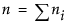:
The power is the probability that the F test of a hypothesis is significant at the α significance level, when the true effect size is a specified value. If the true effect size equals δ, then the test statistic has a noncentral F distribution with noncentrality parameter
The power of the test increases with λ. In particular, the power increases with sample size n and effect size δ, and decreases with error variance σ2.
Some books (for example, Cohen, 1977) use a standardized effect size, Δ = δ/σ, rather than the raw effect size used by JMP. For the standardized effect size, the noncentrality parameter equals λ = nΔ2.
In the Power Details window, δ is initially set to. SSHyp is the sum of squares for the hypothesis, and n is the number of observations in the current study. SSHyp is an estimate of δ computed from the data, but such estimates are biased (Wright and O’Brien, 1988). To calculate power using a sample estimate for δ, you might want to use the Adjusted Power and Confidence Interval calculation rather than the Solve for Power calculation. The adjusted power calculation uses an estimate of δ that is partially corrected for bias. See Computations for the Adjusted Power in Statistical Details.
Plot of Power by Sample Size
The least significant number (LSN) is the smallest number of observations that leads to a significant test result, given the specified values of delta, sigma, and alpha. Recall that delta, sigma, and alpha represent, respectively, the effect size, the error standard deviation, and the significance level.
Note: LSN is not a recommendation of how large a sample to take because it does not take into account the probability of significance. It is computed based on specified values of delta and sigma.
 • If the LSN is less than the actual sample size n, then the effect is significant.
 • If the LSN is greater than n, the effect is not significant. If you believe that more data will show essentially the same structural results as does the current sample, the LSN suggests how much data you would need to achieve significance.
 • If the LSN is equal to n, then the p-value is equal to the significance level alpha. The test is on the border of significance.
 • The power of the test for the effect size, calculated when n = LSN, is always greater than or equal to 0.5. Note, however, that the power can be close to 0.5, which is considered low for planning purposes.
The LSV, or least significant value, is computed for single-degree-of-freedom hypothesis tests. These include tests for the significance of individual model parameters, as well as more general linear contrasts. The LSV is the smallest effect size, in absolute value, that would be significant at level alpha. The LSV gives a measure of the sensitivity of the test on the scale of the parameter, rather than on a probability scale.
 • If the absolute value of the parameter estimate or contrast is greater than or equal to the LSV, then the p-value of the significance test is less than or equal to alpha.
 •
The power of a test is the probability that the test gives a significant result. The power is a function of the effect size δ, the significance level α, the error standard deviation σ, and the sample size n. The power is the probability that you will detect a specified effect size at a given significance level. In general, you would like to design studies that have high power of detecting differences that are of practical or scientific importance.
 • If the true value of the parameter is not the hypothesized value, in general, you want the power to be as large as possible.
 • Power increases as: sample size increases; error variance decreases; the difference between the true parameter value and the hypothesized value increases.
Note that the adjusted power and confidence interval calculations are relevant only for the value of δ estimated from the data (the value provided by default). For other values of delta, the adjusted power and confidence interval are not provided.
This example illustrates a retrospective power analysis using the Big Class.jmp sample data table. The Power Details window (Power Details Window for Age) permits exploration of various quantities over ranges of values for α, σ, δ, and Number, or study size. Clicking Done replaces the window with the results of the calculations.
 1 Select Help > Sample Data Library and open Big Class.jmp.
 2 Select Analyze > Fit Model.
 3 Select weight and click Y.
 4 Add age, sex, and height as the effects.
 5 Click Run.
 6 From the red triangle next to age, select Power Analysis.
Power Details Window for Age
 7 Replace the δ value in the From box with 3, and enter 6 and 1 in the To and By boxes as shown in Power Details Window for Age.
 8 Replace the Number value in the From box with 20, and enter 60 and 10 in the To and By boxes as shown in Power Details Window for Age.
 9 Select Solve for Power and Solve for Least Significant Number.
 10 Click Done.
 11
Power Details Report for Age
Consider a situation where you are comparing the means of three independent groups. To obtain sample sizes to achieve a given power, select DOE > Sample Size and Power and then select k Sample Means. Here, you enter your estimate of the error standard deviation. In the Prospective Means list, you enter means that reflect the smallest differences that you want to detect. If, for example, you want to detect a difference of 8 units between any two means, enter the extreme values of the means, say, 40, 40, and 48. Because the power is based on deviations from the grand mean, you can enter only values that reflect the desired differences (for example 0, 0, and 8).
If you click Continue, you obtain a graph of power versus sample size. If you specify either power or sample size in the Sample Size window, the other quantity is computed. In particular, if you specify power, the sample size that is provided is the total required sample size. The k Sample Means calculation assumes equal group sizes. For three groups, you would divide the sample size by 3 to obtain the individual group sizes. For more information about k Sample Means, see the Design of Experiments Guide book.
Bacteria.jmp Data Table
 • The Group column identifies the groups.
 • The Means column reflects the smallest difference among the columns that it is important to detect. Here, it is assumed that the control group has a mean of about 40. You want the test to be significant if either treatment group has a mean that is at least 8 units higher than the mean of the control group. For this reason, you assign a mean of 48 to one of the two treatment groups. Set the mean of the other treatment group equal to that of the control group. (Alternatively, you could assign the control group and one of the treatment groups means of 0 and the remaining treatment group a mean of 8.) Note that the differences in the group means are population values.
 • The Relative Sizes column shows the desired relative sizes of the treatment groups. This column indicates that the control group needs to be twice as large as each of the treatment groups. (Alternatively, you could start out with an initial guess for the treatment sizes that respects the relative size criterion.)
Note: The Relative Sizes column must be assigned the role of a Freq (frequency). See the symbol to the right of the column name in the Columns panel.
Next, use Fit Model to fit a one-way analysis of variance model (Fit Model Launch Window for Bacteria Study). Note that Relative Sizes is declared as Freq in the launch window. Also, the Minimal Report emphasis option is selected.
Fit Model Launch Window for Bacteria Study
Click Run to obtain the Fit Least Squares report. The report shows Root Mean Square Error and Sum of Squares for Error as 0.0, because you specified a data table with no error variation within the groups. You must enter a proposed range of values for the error variation to obtain the power analysis. Specifically, you have information that the error variation will be about 5 but might be as large as 6.
 1 Click the disclosure icon next to Effect Details to open this report.
 2 From the red triangle menu next to Group, select Power Analysis.
 3 To explore the range of error variation suspected by the scientist, under σ, enter 5 in the first box and 6 in the second box (Power Details Window for Bacteria Study).
 4 Note that δ is entered as 3.464102. This is the effect size that corresponds to the specified difference in the group means. The data table contains three hidden columns that illustrate the calculation of the effect size. (See Unbalanced One-Way Layout.)
 5 To explore power over a range of study sizes, under Number, enter 16 in the first box, 64 in the second box, and an increment of 4 in the third box (Power Details Window for Bacteria Study).
 6 Select Solve for Power.
 7 Click Done.
Power Details Window for Bacteria Study
The Power Details report, shown in Power Details Report for Bacteria Study, replaces the Power Details window. This report gives power calculations for α = 0.05, for all combinations of σ = 5 and 6, and sample sizes of 16 to 64 in increments of size 4. When σ is 5, to obtain about 90% power, you need a total sample size of about 32. You need 16 subjects in the control group and 8 in each of the treatment groups. On the other hand, if σ is 6, then a total of 44 subjects is required.
Power Details Report for Bacteria Study
Power Plot for Bacteria Study